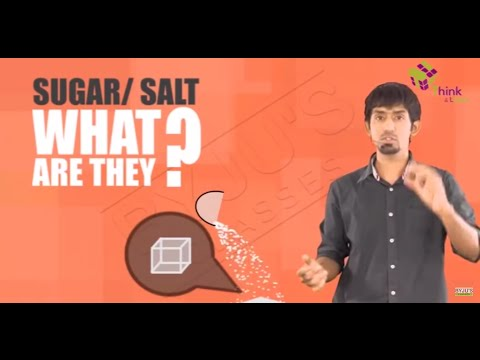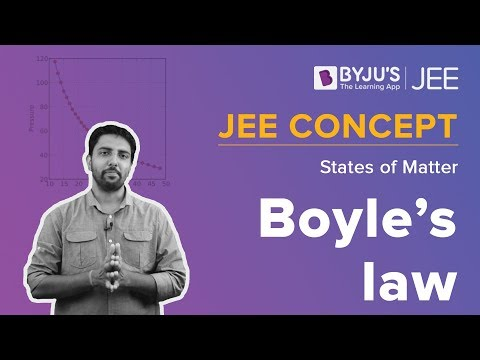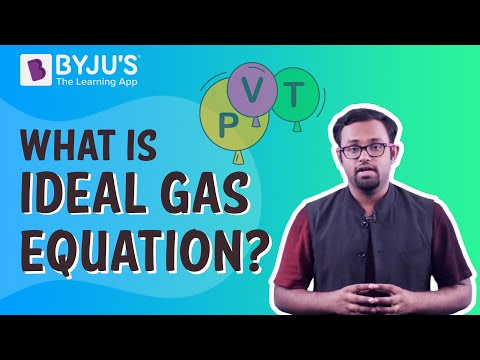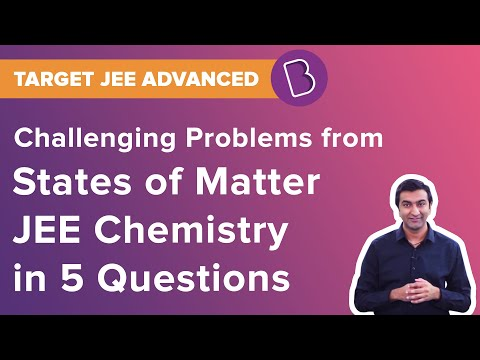# States of Matter Class 11 Notes - Chapter 5

According to the CBSE Syllabus 2023-24, this chapter has been removed from NCERT Class 11 Chemistry textbook.

The intermolecular forces run between the particles of matter. There exists a pure electrostatic force between two ions which are oppositely charged. The intermolecular forces are different from these pure electrostatic forces.Determining the state of matter is done by the competition between intermolecular interactions and thermal energy. Various properties of matter in bulk, such as a change in state, characteristics of liquids and solids, gases behaviour, depend on two factors:

• The interaction type between them
• The energy of constituent particles

The change of state does not affect the chemical properties of a substance, but the change in the physical state affects the reactivity.

## Boyle’s Law

Charles’s Law states that the volume of a given mass of gas is directly proportional to the absolute temperature at constant pressure. It is expressed as PV = k where P is pressure, V is volume, and k is constant. This law is also referred to as the Boyle-Mariotte law or Mariotte’s law.

#### For more information on Boyle’s Law and Ideal Gas Equation, watch the below videos## Charles’ Law

Charles’s Law states that the volume of a given mass of gas is inversely proportional to the absolute pressure at a constant temperature. It is expressed as V/T = k where V is the volume of gas, T is the temperature of the gas, and k is constant. The temperature is measured in the Kelvin scale. This law is also termed the law of volumes.

 Also Access NCERT Solutions for Class 11 Chemistry Chapter 5 NCERT Exemplar for Class 11 Chemistry Chapter 5

### Few Important Questions

1. Given: Quantity of gas = 4.0 mol occupies 5 dm3, Pressure = 3.32 bar, R = 0.083 bar dm3 K-1 mol-1.
2. Calculate the molar mass of phosphorous with the following details:

The quantity of phosphorus vapour is 34.05 mL, and the weight is 0.0625 g. At 0.1 bar pressure and 546 °C temperature.

1. What is the physical importance of van der Waals parameters? Explain.

#### To solve questions on the State of Matter, watch the below videoTo discover more about this chapter, find and browse through this States Of Matter Class 11 Notes offered by BYJU’S.

## Frequently Asked Questions on CBSE Class 11 Chemistry Notes Chapter 5 States of Matter

Q1

### What is Charle’s Law?

Charles’s law states that the volume occupied by a fixed amount of gas is directly proportional to its absolute temperature if the pressure remains constant.

Q2

### What is an ideal gas equation?

The ideal gas equation is formulated as PV = nRT. In this equation, P refers to the pressure of the ideal gas, V is the volume of the ideal gas, n is the total amount of ideal gas that is measured in terms of moles, R is the universal gas constant, and T is the temperature.

Q3

### What is intermolecular space?

Intermolecular spaces can be defined as the space between two molecules or atoms.